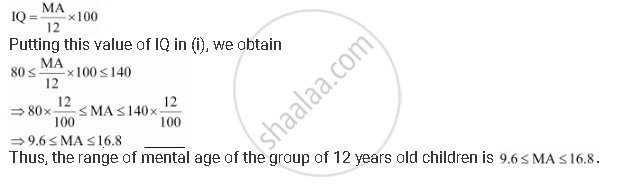CBSE (Arts) Class 11CBSE
Share

# IQ of a person is given by the formula Where MA is mental age and CA is chronological age. If 80 ≤ IQ ≤ 140 for a group of 12 years old children, find the range of their mental age. - CBSE (Arts) Class 11 - Mathematics

ConceptGraphical Solution of Linear Inequalities in Two Variables

#### Question

IQ of a person is given by the formula

IQ = (MA)/(CA) xx100

Where MA is mental age and CA is chronological age. If 80 ≤ IQ ≤ 140 for a group of 12 years old children, find the range of their mental age.

#### Solution

It is given that for a group of 12 years old children, 80 ≤ IQ ≤ 140 … (i)

For a group of 12 years old children, CA = 12 yearsIs there an error in this question or solution?

#### APPEARS IN

NCERT Solution for Mathematics Textbook for Class 11 (2018 to Current)
Chapter 6: Linear Inequalities
Q: 14 | Page no. 132

#### Video TutorialsVIEW ALL 

Solution IQ of a person is given by the formula Where MA is mental age and CA is chronological age. If 80 ≤ IQ ≤ 140 for a group of 12 years old children, find the range of their mental age. Concept: Graphical Solution of Linear Inequalities in Two Variables.
S